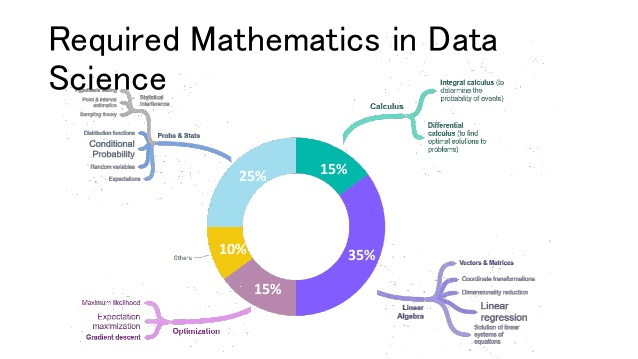# Discrete Maths , Statistics and Probability in Machine Learning

## Use of Descriptive Statistics

Descriptive statistics is a critical concept that every aspiring data scientist needs to learn to understand machine learning when working with classifications like logistic regression, distributions, discrimination analysis, and hypothesis testing.

If you were struggling with Statistics in school then you need to put in your 200 percent to learn the mathematics part of statistics as it is very essential for you to become a successful data scientist. To put it down in simpler words, statistics is the main part of mathematics for machine learning. Some of the fundamental statistics needed for ML are Combinatorics, Axioms, Bayes’ Theorem, Variance and Expectation, Random Variables, Conditional, and Joint Distributions.

## Discrete Maths in Machine Learning

Discrete mathematics is concerned with non-continuous numbers, most often integers. Many applications necessitate the use of discrete numbers. When scheduling a taxi fleet, for example, you cannot send 0.34 taxis; you must send complete ones. You can’t have half a postman or make him visit 1 and a half places to deliver the letters.

Many of the structures in artificial intelligence are discrete. A neural network, for example, has an integer number of nodes and interconnections. It can’t have 0.65 nodes or a ninth of a link. As a result, the mathematics used to construct a neural network must include a discrete element, the integer representing the number of nodes and interconnections.

You can get away with just the fundamentals of discrete math for machine learning unless you wish to work with relational domains, graphical models, combinatorial problems, structured prediction, and so on. To master these concepts you have to refer to books on discrete maths. Luckily for computer science graduates, these concepts are properly covered in their college. However, others may have to put additional efforts to understand this subject. Hence, discrete mathematics is a very important component of AI & ML.## Probability Theory in Machine Learning

To properly work through a machine learning predictive modeling project, it would be reasonable to conclude that probability is essential.

Machine learning is the process of creating prediction models from ambiguous data. Working with faulty or incomplete information is what uncertainty entails.

Uncertainty is crucial to machine learning, yet it is one of the components that creates the most difficulties for newcomers, particularly those coming from a programming background.

In machine learning, there are three major sources of uncertainty: noisy data, limited coverage of the problem area, and of course imperfect models. However, with the help of the right probability tools, we can estimate the solution to the problem.

Probability is essential for hypothesis testing and distributions like the Gaussian distribution and the probability density function.